# Introduction

The corrplot package is a graphical display of a correlation matrix, confidence interval. It also contains some algorithms to do matrix reordering. In addition, corrplot is good at details, including choosing color, text labels, color labels, layout, etc.

# Visualization methods

There are seven visualization methods (parameter `method`) in corrplot package, named `"circle"`, `"square"`, `"ellipse"`, `"number"`, `"shade"`, `"color"`, `"pie"`.

Positive correlations are displayed in blue and negative correlations in red color. Color intensity and the size of the circle are proportional to the correlation coefficients.

``library(corrplot)``
``## corrplot 0.88 loaded``
``````M <- cor(mtcars)
corrplot(M, method = "circle")``````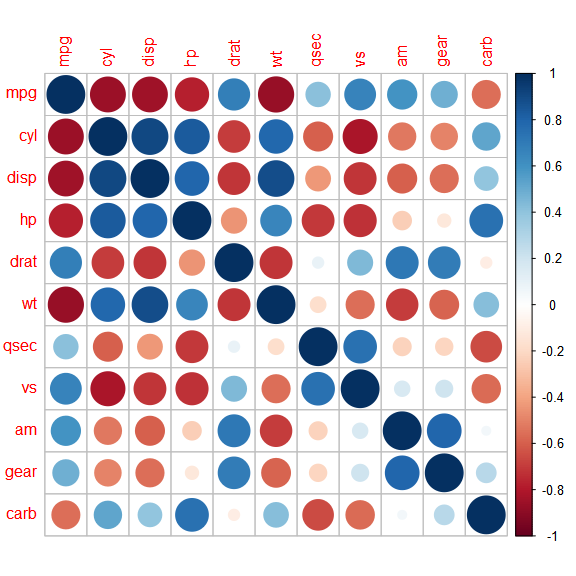``corrplot(M, method = "square")``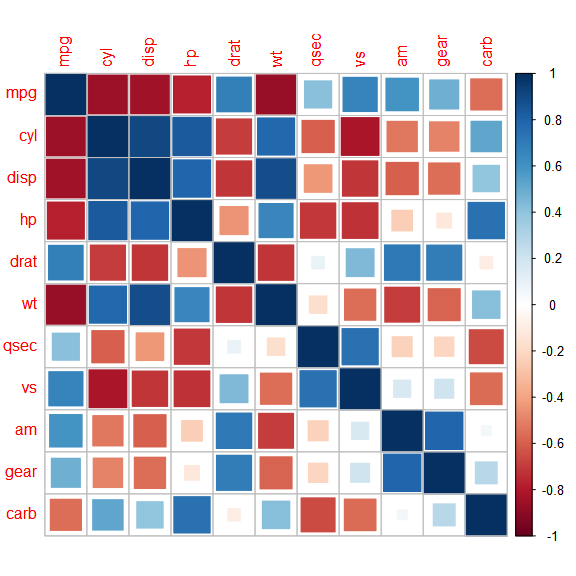``corrplot(M, method = "ellipse")``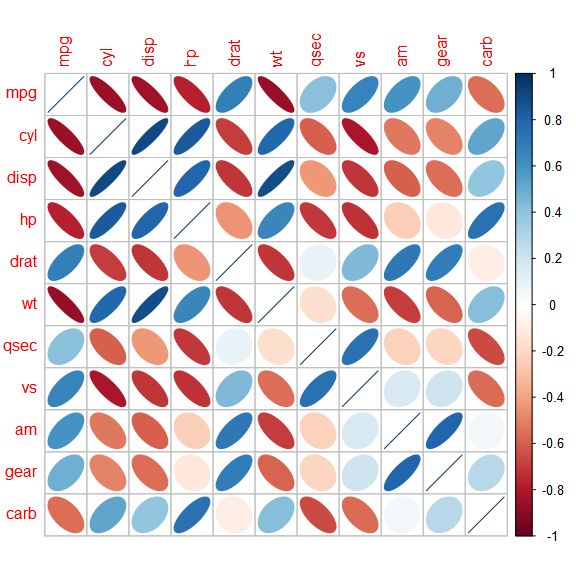``corrplot(M, method = "number") # Display the correlation coefficient``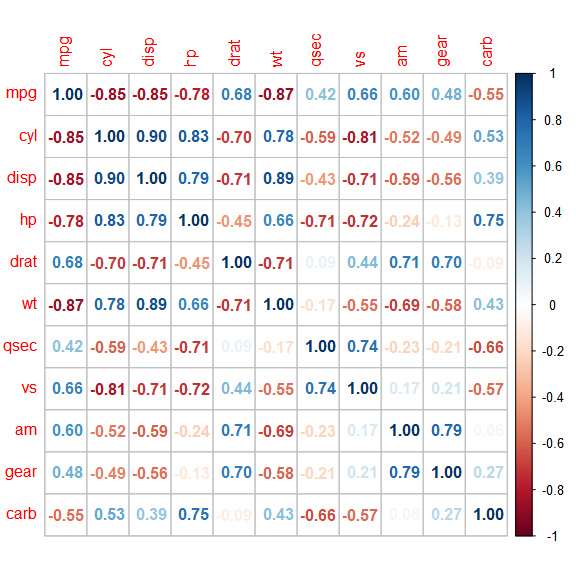``corrplot(M, method = "shade")``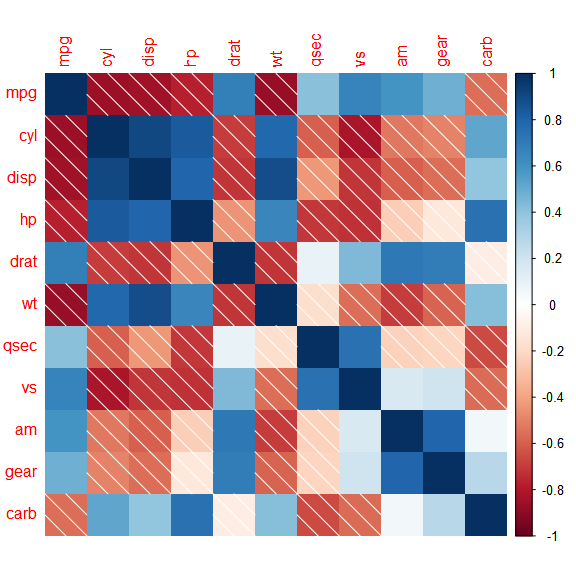``corrplot(M, method = "color")``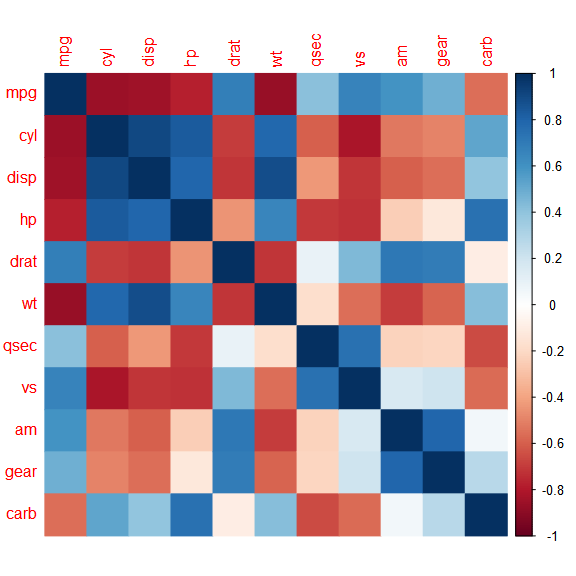``corrplot(M, method = "pie")``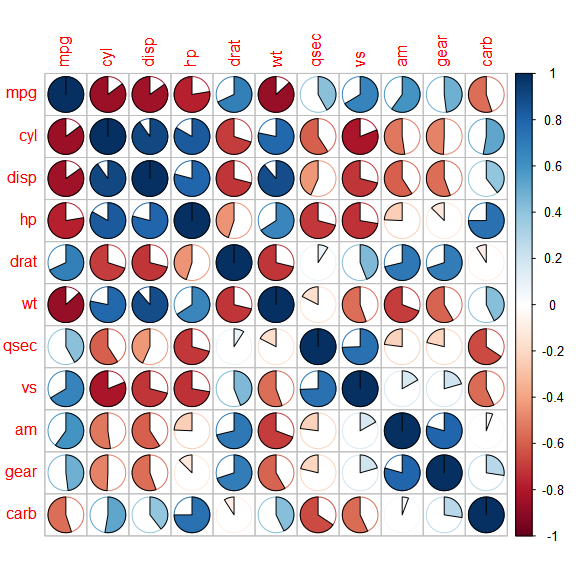# Layout

There are three layout types (parameter `type`): - `"full"` (default) : display full correlation matrix - `"upper"` : display upper triangular of the correlation matrix - `"lower"` : display lower triangular of the correlation matrix

``corrplot(M, type = "lower")``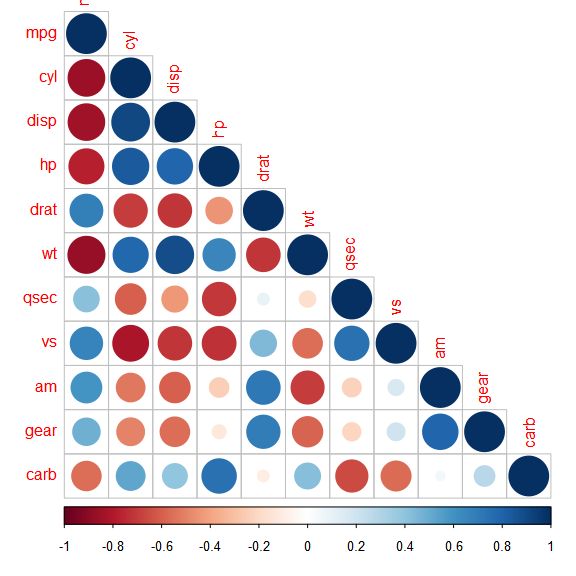`corrplot.mixed()` is a wrapped function for mixed visualization style.

``corrplot.mixed(M)``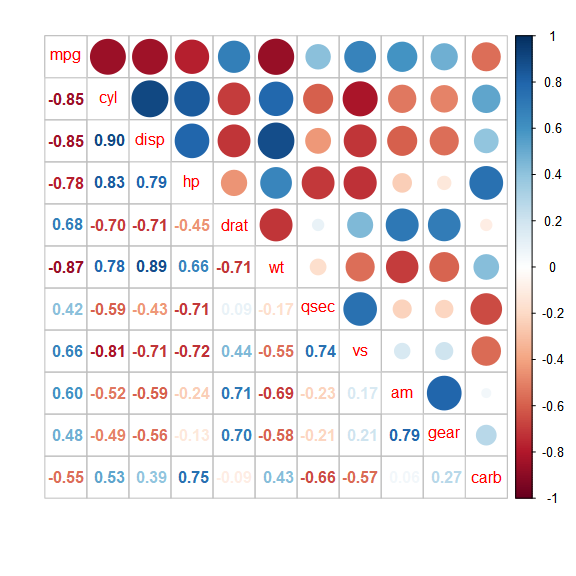``corrplot.mixed(M, lower.col = "black", number.cex = .7)``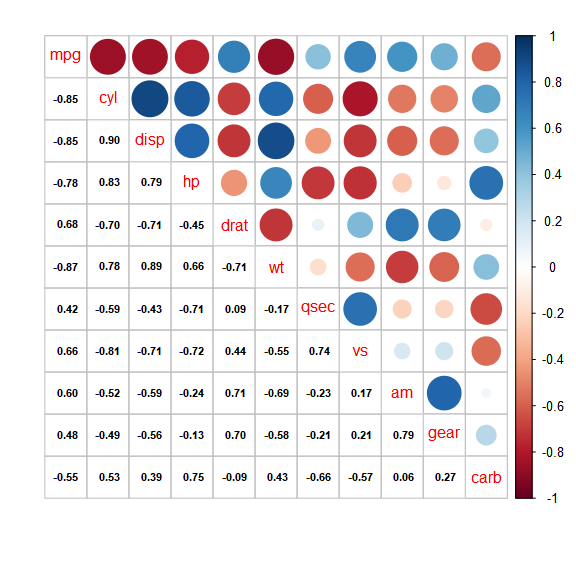``corrplot.mixed(M, lower = "square", upper = "circle", tl.col = "black")``